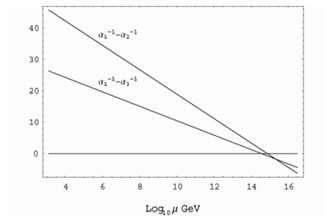### RESEARCH SPOTLIGHT

Particle Physics from a Warped Extra Dimension
By: Kaustubh Agashe

The Standard Model of particle physics has been developed over the past couple of decades to address the fundamental questions: what is our universe made of and what holds its constituents together? Within this model, the basic building blocks of nature (the elementary particles) are of two kinds: (1) matter particles called quarks (which make up protons and neutrons and hence most of the ordinary matter) and leptons (such as the electron) and (2) force carriers which mediate interactions between the matter particles,namely, the electromagnetic force (ordinary light); the weak force (which appears in radioactivity); and the strong force (which is involved in nuclear reactions). In addition, there is the force of gravity which is responsible for holding us to the earth and, in turn, the earth to the Sun. However, in spite of its success in describing the observed interactions of elementary particles, the Standard Model is an incomplete theory since it leaves many questions unanswered. For example, why is there such a big hierarchy of quark and lepton masses (the electron is a million times lighter than the top quark, which is the heaviest known quark)? Or, why are the strengths of the various forces so disparate? Specifically, the mass of carriers of the weak force is about 10,000 trillion times smaller than the mass scale of gravity (which is known as the Planck scale). Such a huge hierarchy in mass scales is required in order to account for the feebleness of gravity compared with the other forces. Another mystery is that most of matter in the universe seems to be “dark”, i.e., it is not made of ordinary matter.

In 1999, Lisa Randall and Raman Sundrum proposed a novel extension of the Standard Model to solve the Planck-weak mass scale hierarchy problem. Their set-up is based on “warped” 5th dimension, where the mass scales at the two ends of the extra dimension are vastly different due to the presence of the curvature in the extra dimension. So, if the gravitational physics resides at one end of the extra dimension, whereas the physics of weak force occupies the other end, then it is natural for the mass scales of gravity and the weak force to be hierarchical. It was also realized that profiles for quarks and leptons in the same extra dimension can account for the hierarchies in their masses. Thus, the upshot is that there is a geometrical origin for all hierarchies of Standard Model: the Planck weak mass scales and that of quark and lepton masses.FIG. 1: Evolution of the strengths of the 3 forces in the warped extra dimensional model with energy.

Over the past few years, I have been involved in detailed studies of various aspects of the Randall-Sundrum model. In this model, there are excitations of the Standard Model particles in the 5th dimension which are called Kaluza-Klein particles. I have discovered mechanisms within this framework whereby masses of these Kaluza-Klein particles as small as a 1000 times the mass of the proton are allowed by the current experimental data, whereas previous models required the mass scale to be much larger for this consistency. I also showed that these particles will be directly accessible to an upcoming high energy experiment known as the Large Hadron Collider in Europe. Moreover, indirect effects of these new particles will be seen in other experiments probing the properties of the quarks and leptons. One of the holy grails of particle physics is grand unification which is the idea that the 3 forces (other than gravity) are different aspects of the same interaction. As mentioned above, the strengths of the 3 forces are observed to be quite different when measured at low energies, but these strengths evolve as we go to higher energies so that it is possible that the strengths are the same at some very high energy scale. However, in the Standard Model, such a unification of the 3 forces does not occur. We demonstrated that in the Randall-Sundrum model the evolution of the strengths of these forces is modified relative to that in
the Standard Model due to profiles for quarks and leptons in the 5th dimension, resulting in a precise meeting of the strengths of the forces: see Figure 1. In addition, the above grand unified model has a particle which can play the role of dark matter of the universe, with good prospects to be seen in ongoing direct searches for dark matter. Thus, my work has shown that the framework of a warped extra dimension solves all the above-mentioned puzzles of the Standard Model. Moreover, these ideas will soon be tested.

--------------------------------------------------------------------------------------------------------------------------------------------------------------------------------------------
Dr. Agashe is an Assistant Professor at the University of Maryland Department of Physics. He is a member of the Elementary Particle Group. For questions and comments, please contact him at kagashe@umd.edu.